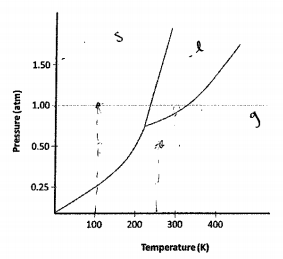# Problem: The phase diagram for compound X is shown above. You wish to purify a sample of X which was collected at P = 1.0 atm and T = 100 K by subliming it. In order to sublime the sample, you should:(a) Increase P to 1.5 atm and then increase T to 300 K(b) Increase T to 300 K, keeping P at 1.0 atm(c) Lower P to 0.5 atm and then increase T to 250 K(d) Increase T to 300 K and then lower P to 0.5 atm(e) the sample cannot be sublimed

###### FREE Expert Solution

Analyze the given phase diagram and figure out how to sublime compound X starting at 1 atm, 100K

Sublimation is the process of converting solid to gas. This means we have to directly convert solid to gas without passing to the liquid phase

The red line below is the transition of solid to gas###### Problem DetailsThe phase diagram for compound X is shown above. You wish to purify a sample of X which was collected at = 1.0 atm and = 100 K by subliming it. In order to sublime the sample, you should:

(a) Increase P to 1.5 atm and then increase T to 300 K

(b) Increase T to 300 K, keeping P at 1.0 atm

(c) Lower P to 0.5 atm and then increase T to 250 K

(d) Increase T to 300 K and then lower P to 0.5 atm

(e) the sample cannot be sublimed